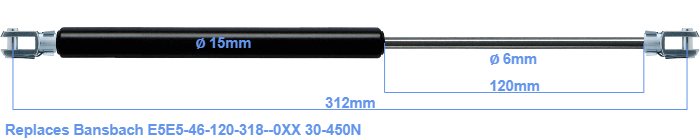# Replacement for Bansbach E5E5-46-120-318–0XX 30-450N

£28.76 (excl. VAT)

Replacement gas spring for the Bansbach E5E5-46-120-318--0XX 30-450 Newton. The fork has a hole diameter of 5.1mm. Brand: Hahn.

Please note:
This replacement gas spring is 6mm shorter than the original gas spring. In general, this will not cause any problems.
 Force Choose an option30 Newton40 Newton50 Newton60 Newton80 Newton100 Newton120 Newton140 Newton150 Newton160 Newton180 Newton200 Newton220 Newton240 Newton250 Newton260 Newton280 Newton300 Newton320 Newton340 Newton350 Newton360 Newton380 Newton400 Newton420 Newton440 Newton450 NewtonClear